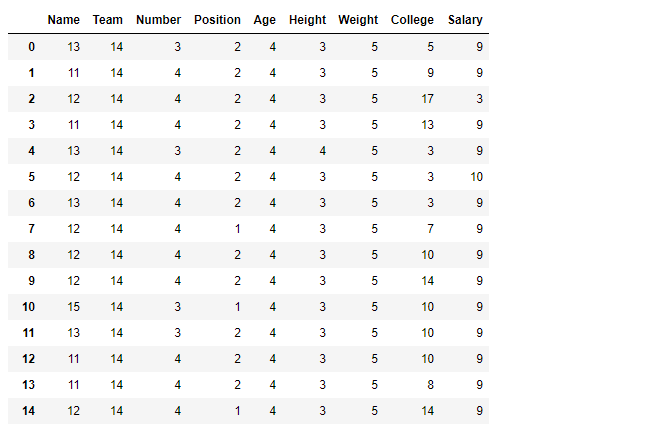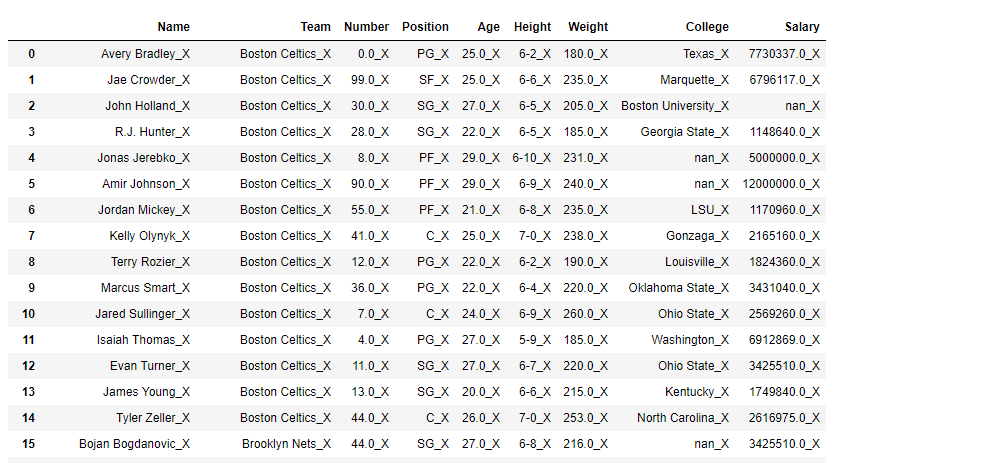Related Articles

# Python | Pandas dataframe.applymap()

• Last Updated : 16 Nov, 2018

Python is a great language for doing data analysis, primarily because of the fantastic ecosystem of data-centric python packages. Pandas is one of those packages and makes importing and analyzing data much easier.

`Dataframe.applymap()` method applies a function that accepts and returns a scalar to every element of a DataFrame.

```Syntax: DataFrame.applymap(func)

Parameters:
func: Python function, returns a single value from a single value.

Returns: Transformed DataFrame.
```

Example #1: Apply the `applymap()` function on the dataframe to find the no. of characters in all cells.

 `# importing pandas as pd``import` `pandas as pd`` ` `# Making data frame from the csv file``df ``=` `pd.read_csv(``"nba.csv"``)`` ` `# Printing the first 10 rows of ``# the data frame for visualization``df[:``10``]``# Using lambda function we first convert all ``# the cell to a string value and then find``# its length using len() function``df.applymap(``lambda` `x: ``len``(``str``(x)))`

Output:Notice how all nan value has been converted to string nan and their length is evaluated to be 3.

Example #2: Append `_X `in each cell using `applymap()` function.

In order to append `_X `in each cell, first convert each cell into a string.

 `# importing pandas as pd``import` `pandas as pd`` ` `# Making data frame from the csv file``df ``=` `pd.read_csv(``"nba.csv"``)`` ` `# Using applymap() to append '_X'``# in each cell of the dataframe``df.applymap(``lambda` `x: ``str``(x) ``+` `'_X'``)`

Output:Attention geek! Strengthen your foundations with the Python Programming Foundation Course and learn the basics.

To begin with, your interview preparations Enhance your Data Structures concepts with the Python DS Course. And to begin with your Machine Learning Journey, join the Machine Learning – Basic Level Course

My Personal Notes arrow_drop_up